Radiation Pressure Induced Mechanical Vibration of Optical Microdisk Oscillators

Overview

This report focuses on the experimental observation of mechanical oscillations in optical microcavities, induced by radiation pressure in ultra-high-Q optical microdisk cavities (we refer to refs. [1-6]). The coupling of energy back and forth from the optical to the mechanical domain gives rise to a so-called parametric oscillation in which a steady build-up of mechanical vibration occurs when an optical threshold energy has been surpassed.

Mechanical Properties of Microdisk Oscillators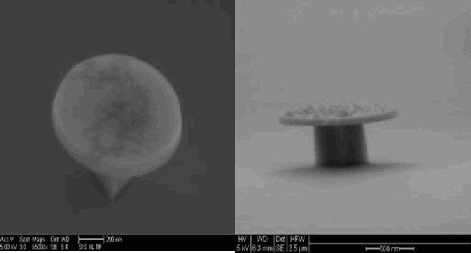Fig. 1: SEM micrographs of optical microdisk oscillators (K. De Greve, 2007-05-29).

Optical microdisk oscillators, as shown in Fig. 1, are essentially semiconductor/dielectric disks of sizes in the micrometer-regime. They have been extensively used over the last 20 years in the fields of micro/nanophotonics and quantum optics (see  for a thorough review of the field of optical oscillators). Beyond their remarkable optical properties, which will be briefly discussed in the next section, they are also interesting from a mechanical point of view, especially when actuated in their vibrational eigenmodes.

When a microdisk oscillator interacts with a radially symmetric mechanical force F(t) (cf. Fig. 2a), it will expand resp.contract under the influence of that force and its effective stiffness, Keff.

In view of the radial symmetry, an effective 1D-model can be developed, as shown in Fig. 2b, which can also account for the dynamic properties of such a system under the influence of the time-dependent force F(t):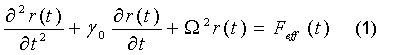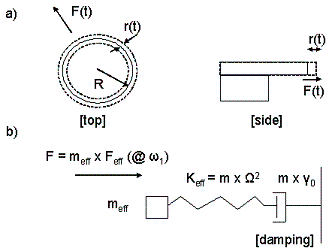Fig. 2: Radially symmetric mechanical vibration of a microdisk resonator (a) and its 1D equivalent model (b).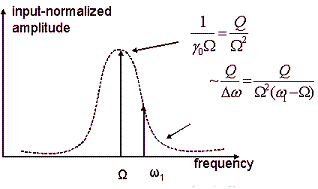Fig. 3: Lorentzian lineshape, amplitude response of a driven, damped harmonic oscillator.

in which r(t) stands for the deviation form equilibrium (the radial displacement), Feff (F/meff) for the effective mass-renormalized actuation force, γ0 for a mass-renormalized friction constant, and Ω =(Keff/meff)1/2, the ratio of the effective stiffness constant Keff and the effective mass meff) is the resonance frequency of the system.

When driven by an oscillatory excitation at frequency ω1 (possibly detuned from the resonance frequency Ω), the steady state response of the system, after an initial transient, will be at the driving frequency ω1: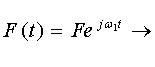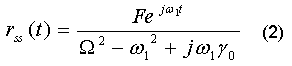which comes down to the well-known Lorentzian response, consisting of both a phase and amplitude part, the amplitude of which peaks at ω1 = Ω as shown in Fig. 3. Note that we introduced in that figure the constant Q (=Ω/γ0), commonly denoted as quality factor. From the Lorentzian lineshape, the full width at half maximum Δω equals Ω/Q, defining an effective bandwidth of the system.

Optical Properties of Microdisk Oscillators

The operation principle of optical microdisk oscillators is described in Fig. 4. Through evanescent coupling, light from a waveguide is coupled into the microdisk, in which (lossless) total internal reflection confines the light within the microdisk. For frequencies ω0 equalling c/nR, with R the radius of the microdisk, the circulating light constructively interferes, leading to an effective build-up of optical energy.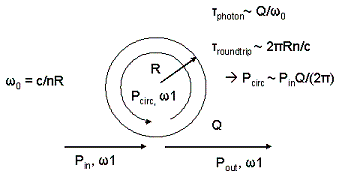Fig. 4: Operation principle of optical microdisk oscillators. Pin resp. Pout represent the incoming resp. outgoing optical power. Pcirc is the circulating optical power, n is the index of refraction and Q is the total quality factor of the resonator, taking into account input/output and intrinsic losses.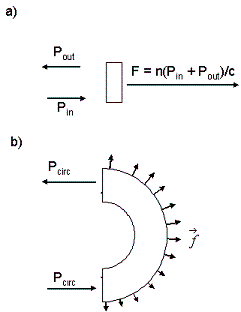Fig. 5: a) Radiation-pressure upon reflection from a wall. Note that in a lossless system, Pout = Pin, hence F = 2nPin/c. b) Radiation pressure in microdisk resonators. f is the (effective-mass renormalized) force per unit length. Pcirc is the circulating optical power (PinQ/2π).

The energy build-up due to the incoupling of light from the input waveguide is eventually balanced by the losses in the system, described by an effective quality factor Q (note that as the losses due to intrinsic absorption and input/output coupling can be considered additive to first order, the effective quality factor Q equals (Qint-1 + Qin-1 + Qout-1)-1). Advances in micro- and nanofabrication have led to Q factors on the order of 108 to be realized experimentally . By equating the losses from a circulating optical power Pcirc to the (net) input power, an effective increase of the circulating power Pcirc over the input power Pin of Q/2π (> 107) can be realized.

When excited with incoming light at frequency ω1, the transmitted output power will show an inverse Lorentzian lineshape defined by a linewidth Δω = ω0/Q, which for optical frequencies, and with Q-factors in the 107-108 range leads to linewidths in the (tens of) MHz range.

Radiation Pressure in Microdisk Cavities.

From Einstein's theory of relativity, we know that a photon with energy E will have a momentum p = E/c. When reflecting off a surface, a net momentum change of pout - pin is exerted on the photon. In view of Newton's second law, an equal and opposite momentum change will be exerted on the wall. Without losses, pout = -pin, and the net impulse on the wall will be 2pin = 2Ein/c.

A light field impinging on a wall can be regarded as a stream of photons bouncing into and off that wall. When the incoming optical power Pin contains photons of energy E, the number of photons impinging per second equals Pin/E. As the net impulse (per photon) exerted on the wall is equal to 2E/c, the total impulse exerted on the wall per unit time will be 2nPin/c (we account for a reduced speed of light by an index of refraction n). By definition, the net impulse per unit time equals the force exerted on the wall (see Fig. 5 a)).

In optical microdisk oscillators, total internal reflection confines the light inside the oscillator, effectively bouncing the photons off the sidewall of the oscillator. Upon circulating halfway through the oscillator, there is a net force on the sidewall, equal to the integrated force f per unit length (in view of the radial symmetry, this per-unit-length force is uniform over the entire oscillator, see Fig. 5 b)).  From this, the force per unit legth can be calculated. Integrating this force over the entire boundary, and renormalizing to the effective mass of the oscillator, the total force F equals :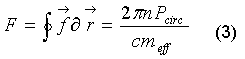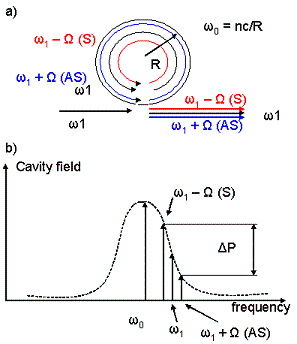Fig. 6: a) the optical field frequencies in a microdisk in the case of mechanical oscillation at frequency Ω. The input frequency is ω1. Stokes (ω1 - Ω) and anti-Stokes sidebands (ω1 - Ω) will oscillate within the cavity. b) The respective optical frequencies displayed together with the Lorentzian lineshape in the microdisk. Figure redrawn from .

in which meff is the effective mass of the microdisk, and Pcirc the circulating optical power (amplified by a factor of ~ Q/(2π) over the input power Pin). In view of the Q/(2π) amplification, the circulating optical power can reach values on the order of 100W for input powers in the mW regime , and the total force exerted on the walls (this time non-mass-normalized) 10-5 N. With these force levels, mechanical effects caused by the circulating optical power cannot be neglected, as will be shown below.

Radiation-Pressure Induced Mechanical Oscillation in Microdisk Oscillators.

Heuristic Approach

An oscillating circulating optical field intensity will give rise to an oscillating radiation pressure on the sidewalls of the optical microdisks. When the oscillation frequency falls approximately within the mechanical resonance bandwidth, a significant mechanical vibration will be excited.

Conversely, a changing effective radius of the optical microdisk will shift the resonance frequency according to the formula ω0(t)=c/n(R+r(t)), where r(t) denotes the deviation from equilibrium of the microdisk radius.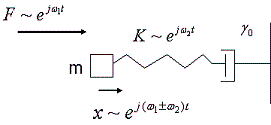Fig. 7: The classical textbook example of parametric oscillation:a mass attached to a spring, driven at frequency ω1  has a spring constant K oscillating at frequency ω1 . The resulting oscillation of the mass-spring system will have frequency components ω1 +ω2  resp. ω1 -ω2 .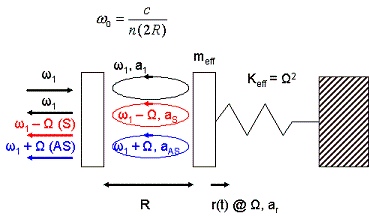Fig. 8: 1D, Fabry-Perot-resonator based effective model for the parametric oscillation. Ω, ω1, ω1-Ω, ω1+Ω are the mechanical, optical input, Stokes and anti-Stokes frequencies; ar, a1, aS and aAS are the normalized time-dependent field amplitudes of the mechanical, input optical and Stokes/anti-Stokes waves. Figure adapted from .

A mechanical oscillation of the microdisk at frequency Ω leads to a periodically varying input coupling of the incoming light wave (note that the cavity field lineshape is Lorentzian, and centered around ω0; intuitively, one could say that this Lorentzian lineshape will oscillate around ω0 at frequency Ω) and hence a periodically varying circulating optical power. Note the interdependence of these two phenomena: a mechanical oscillation leads to an optical power oscillation, and vice versa.

Although the physical description is more complicated than this intuitive reasoning, a fairly elucidating view on this phenomenon involves the corpuscular theory of light. An input photon of frequency ω1 is transformed to respectively a red-shifted, Stokes-photon (ω1-Ω) or a blue-shifted anti-Stokes photon (ω1+Ω) (see Fig. 6a. As long as the Stokes and anti-Stokes photons fall within the optical bandwidth of the oscillator, their amplitudes will be non-neglegible. The beating of the Stokes and anti-Stokes modes with the fundamental leads then to an effective force at frequency Ω.

Blue-detuning of the input frequency ω1 with regard to the (unmodulated) cavity frequency ω01 > ω0), in combination with the Lorentzian lineshape (we refer to Fig. 6b guarantees net power flow ΔP from the optical domain to the mechanical domain, which is subsequently absorbed by the effective mechanical loss mechanisms. We note that, conversely, red detuning of the input wave should then lead to net absorption of mechanical energy, or cooling, as was indeed shown in .

Parametric Oscillation in Optical Microdisk Oscillators

The phenomenon of coupled mechano-optical oscillations is a classic example of the well-known parametric oscillation process (see e.g  for a thorough description of parametric processes in the non-linear optical domain). In the textbook example hereof, a sinusoidally (frequency ω1) varying parameter of a harmonic oscillator leads to a modulation of the oscillator response at frequencies ω12 resp. ω12, as illustrated in Fig. 7. In our system, the optical oscillation can be considered as the harmonic oscillator, and its modulation due to the mechanical vibration as the time-varying spring.

In view of the radial symmetry, an effective 1-D model can be invoked for the parametric oscillation, as developed in  and reproduced in Fig. 8. There, a Fabry-Perot oscillator is considered, where the recircurculation of the optical wave after bouncing back and forth from two mirrors (one semi-transparent: the input mirror) leads to the constructive interference and optical field build-up, similar to the case of optical microdisk cavities. One of the mirrors is attached to a spring, leading to the possibility of parametric oscillation due to mechanical vibration of one mirror. Apart from the type of optical resonator, this is exactly the mechanism of the parametric oscillation in the optical microdisk oscillators.

The slowly varying envelope approximation can be invoked, in which a slow variation of the field amplitudes is decoupled from the fast, oscillatory movement at the respective sinusoidal frequencies. This yields the following equations for the fields (4)-(7):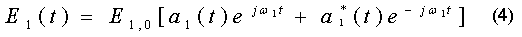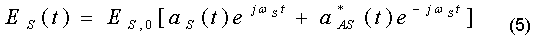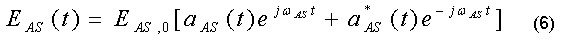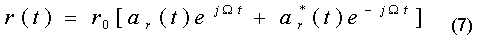Here, a1, aS, aAS and ar are the normalized time-dependent field amplitudes of the input optical, Stokes/anti-Stokes and mechanical waves. Neglecting the anti-Stokes component (justified in the case of blue-detuning, where the anti-Stokes amplitude can be significantly smaller than the Stokes amplitude, cf. Fig. 6, we obtain the following equations for the Stokes and mechanical waves :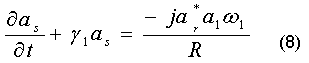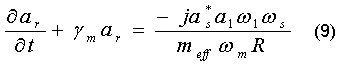where γ1 and γm are the damping coefficients of the optical (and Stokes) vibration and the optical field respectively, and R represents the length of the Fabry-Perot resonator.  Apart from numerical factors, these same equations govern the behavior of the mechanical  and optical oscillations in optical microdisk oscillators (cf. ).

Solutions of the coupled system of eqs. (8) and (9) can be found :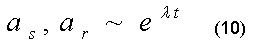leading to a growing amplitude (eventually counteracted by non-linearities in the system, not-considered in this model) as of a threshold circulating power Pth: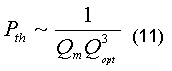Experimental Confirmation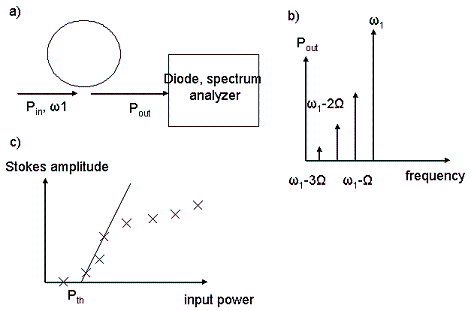Fig. 9: a) Experimental setup, from . b) Detected output power, redrawn from . c) Threshold behavior of the Stokes mode, redrawn from .

The foregoing has been experimentally confirmed in ultra-high Q (106-107) silica optical microdisk oscillators (see refs.  to ). By melting the silica microdisks under illumination with a high-power CO2 laser, an ultrasmooth surface can be created, significantly reducing the surface scattering and associated losses .

The spectral analysis of the transmitted optical power Pout was performed through electrical spectral analysis of the output of a photodiode, in which the inherent quadratic non-linearity leads to beat notes which are detected in an electrical spectrum analyzer . We refer to figs. 9 a) and 9 b). Note that, besides the Stokes sideband, also higher harmonics were observed, due to the non-linear transmission of the microdisk oscillator .

In Fig. 9c, the threshold behavior of the Stokes field amplitude and the mechanical oscillation can be observed.

In order to verify the dependence of the threshold power on the mechanical quality factor as described by equation (11), a setup was designed in which a microprobe was gradually pressed onto the microdisks . By pressing the probe onto the microdisk, the damping of the mechanical vibration and hence the mechanical quality factor could be gradually changed, as shown in Fig. 10a.

A clear linear dependence could be observed of the threshold power on the inverse of the mechanical quality factor, effectively confirming the predicted result of eq. (11), and ruling out other possible parametric oscillation mechanisms besides mechanical vibrations .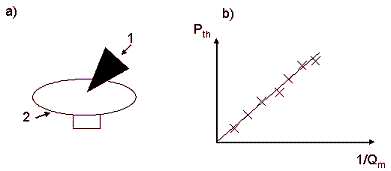Fig. 10: a) Experimental setup for detecting the mechanical quality-factor dependence of the threshold power, adapted from . A mechanical probe (1) is pressed against the microdisk (2), yielding mechanical loss depending on the contact force. b) Threshold power vs. mechanical quality factor, redrawn from .

Conclusion

The study of joint mechanical-optical oscillations, made possible through the creation of ultrahigh-Q optical microdisk oscillators, has confirmed the possibility of radiation pressure effects leading to parametric oscillations in optical microdisks.

Besides the results discussed in this report, other intriguing effects such as optical cooling of of microdisk oscillators have been reported (see ); on the technological side, it has been proposed to use this parametric effect in order to fabricate all-optical clocks (see  and references therein).

© 2007 Kristiaan De Greve. The author grants permission to copy, distribute and display this work in unaltered form, with attribution to the author, for noncommercial purposes only. All other rights, including commercial rights, are reserved to the author.

References

 T. Carmon, H. Rokhsari et al., Phys. Rev. Lett. 94, 223902 (2005).

 T.J. Kippenberg et al., Phys. Rev. Lett. 95, 033901 (2005).

 H. Rokhsari et al., Opt. Express 13, 5293 (2005).

 H. Rokhsari, Appl. Phys. Lett. 89, 261109 (2006).

 A. Schliesser et al., Phys. Rev. Lett. 97, 243905 (2006).

 M. Hossein-Zadeh et al., Phys. Rev. A 74, 023813 (2006).

 V.B. Braginsky et al., Phys. Lett. A 287, 331 (2001).

 K. Vahala, Nature 424, 839 (2003).

 R. Boyd, Non-linear optics, 2nd ed. (Academic Press, 2003).